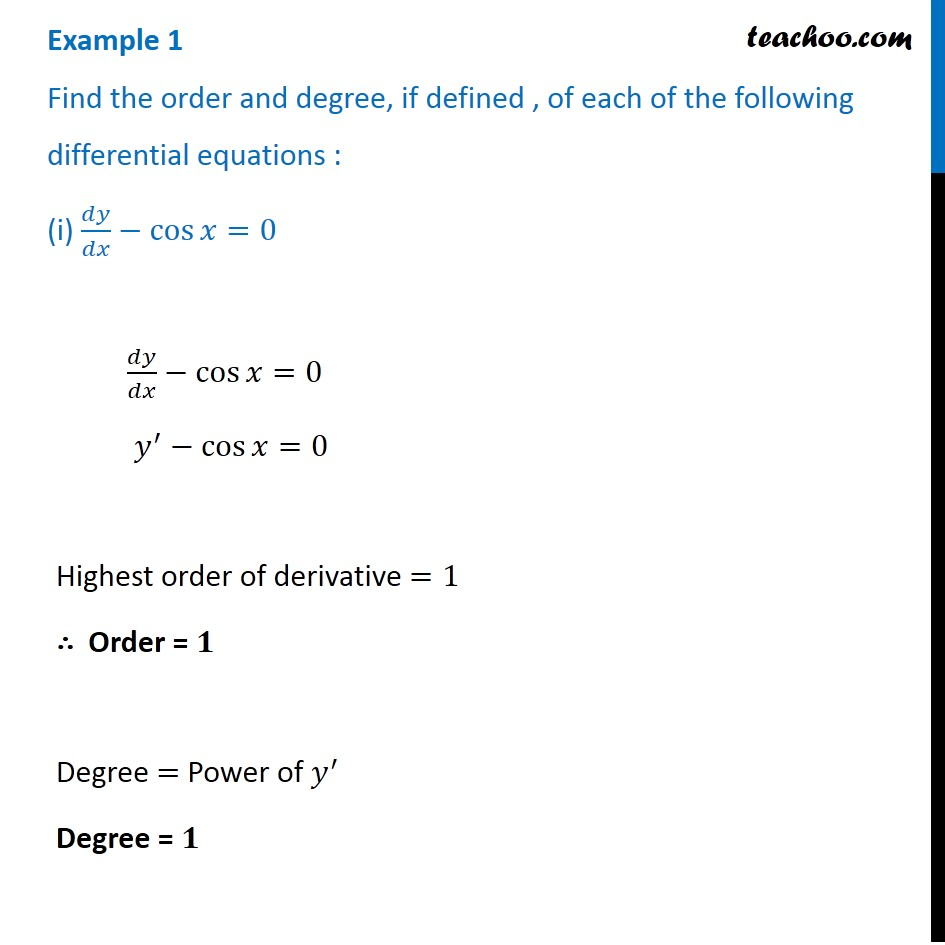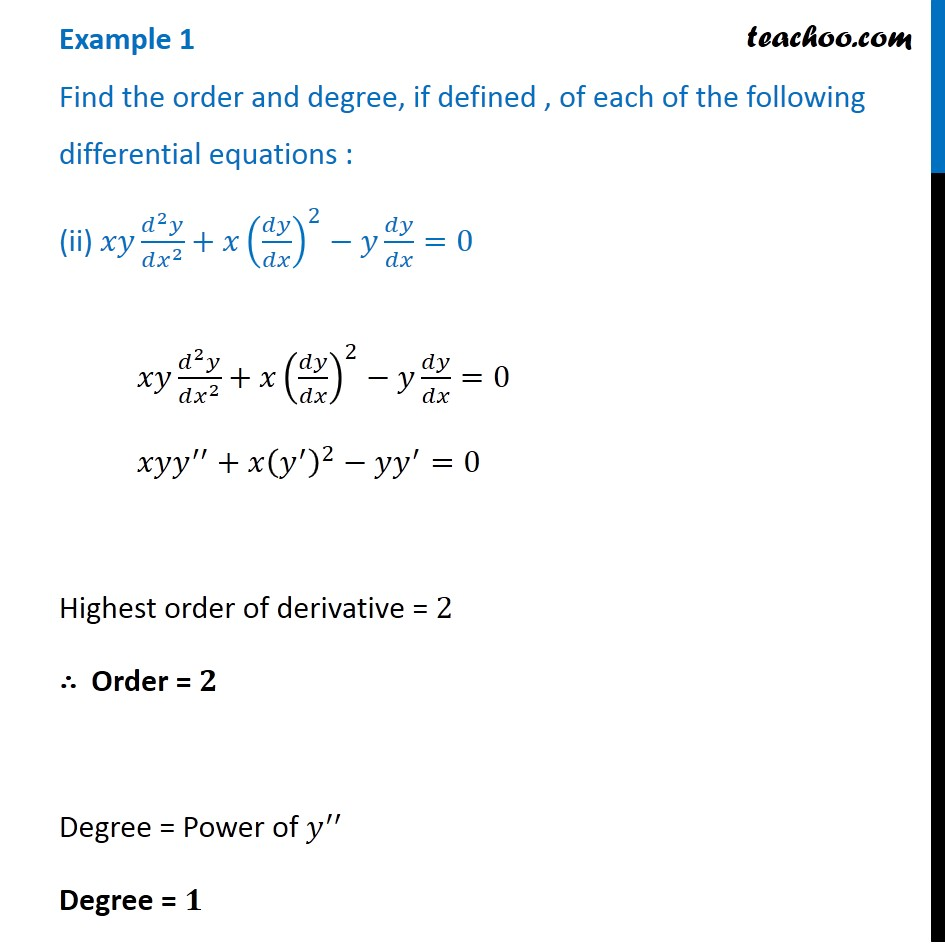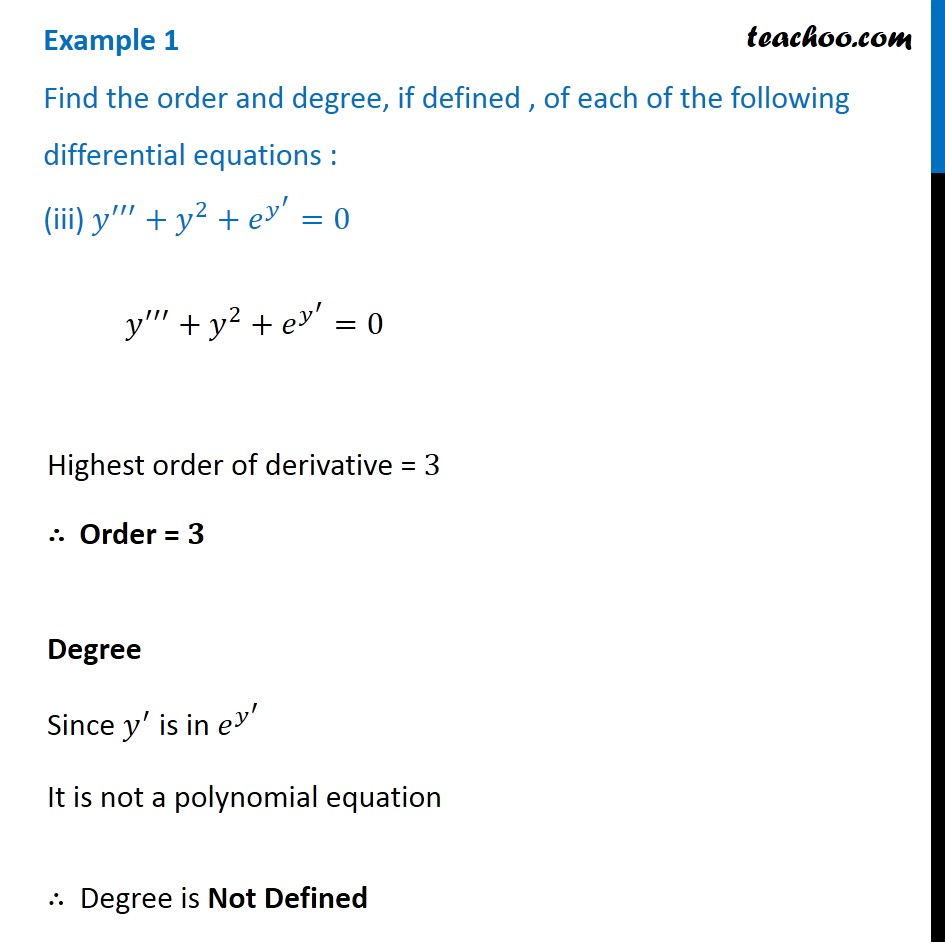Subscribe to our Youtube Channel - https://you.tube/teachoo

1. Class 12
2. Important Questions for exams Class 12
3. Chapter 9 Class 12 Differential Equations

Transcript

Example 1 Find the order and degree, if defined , of each of the following differential equations : (i) 𝑑𝑦/𝑑𝑥−cos⁡〖𝑥=0〗 𝑑𝑦/𝑑𝑥−cos⁡〖𝑥=0〗 𝑦^′−cos⁡〖𝑥=0〗 Highest order of derivative =1 ∴ Order = 𝟏 Degree = Power of 𝑦^′ Degree = 𝟏 Example 1 Find the order and degree, if defined , of each of the following differential equations : (ii) 𝑥𝑦 (𝑑^2 𝑦)/(𝑑𝑥^2 )+𝑥(𝑑𝑦/𝑑𝑥)^2−𝑦 𝑑𝑦/𝑑𝑥=0 𝑥𝑦 (𝑑^2 𝑦)/(𝑑𝑥^2 )+𝑥(𝑑𝑦/𝑑𝑥)^2−𝑦 𝑑𝑦/𝑑𝑥=0 𝑥𝑦𝑦^′′+𝑥(𝑦^′ )^2−𝑦𝑦^′=0 Highest order of derivative = 2 ∴ Order = 𝟐 Degree = Power of 𝑦^′′ Degree = 𝟏 Example 1 Find the order and degree, if defined , of each of the following differential equations : (iii) 𝑦^′′′+𝑦^2+𝑒^(𝑦^′ )=0 𝑦^′′′+𝑦^2+𝑒^(𝑦^′ )=0 Highest order of derivative = 3 ∴ Order = 𝟑 Degree Since 𝑦^′ is in 𝑒^(𝑦^′ ) It is not a polynomial equation ∴ Degree is Not Defined

Chapter 9 Class 12 Differential Equations

Class 12
Important Questions for exams Class 12